“AHEM! There's SAND on my boots!”

CS143 / Project 3 / Scene Classification

In this project we implement scene classification using the bags of words model. For each scene, a set of features is extracted and group them to create a visual vocabulary. Using this visual vocabulary, we then define a histogram for each scene type and use thse histograms coupled with an SVM to classify input scenes into one of several types (suburb, kitchen, industrial, etc.).

The dataset used for this project is the 15 scene (category) database introduced by Lazebnik et al. 2006.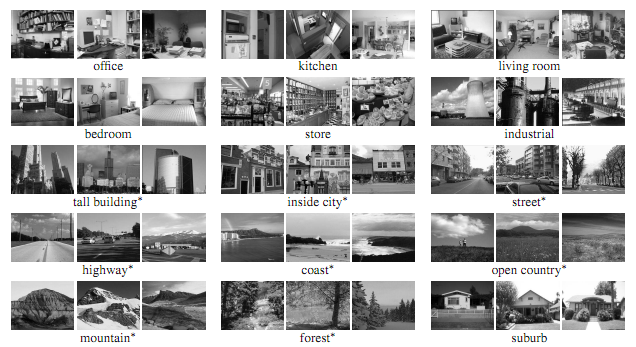0 / Pipeline

In the training phase, dense SIFT features are first extracted from each training image and then clustered using kmeans. Since the total number of features can be quite large, a random subset of ~100k features are chosen from the original set before beinning clustering. While the randomized feature selection decreased recoginition perfomance, the improvement in speed balanced the loss out. The number of clusters, k, was varied depending on the desired vocabulary size, but k = 800 to 1600 tended to yield the best results for the dataset.

Once the vocabulary is formed, histograms of vocabularies for each of the training images are extracted. These histograms are then used to train multiple one vs all SVM scene classifiers.

In the test phase, histograms of the vocabulary are created for each of the test images, and then classified as one of several scene types using the multiclass support vectors. Performance is evaluated using a confusion matrix. The diagonals represent the correct classification rate, accuracy is determined by taking the mean of the diagonals.

1 / Results and Extensions

Instead of focusing on evaluating and trying to improve performance of the classifier, for this project, I focused on evaluating the performance of the classification over varying kernels, kernel parameters, and vocabulary sizes. The classification using bags of words was evaluated over several different vocabulary sizes and different SVM kernels. Vocabulary sizes included [10 20 50 100 200 400 800 1600 3200]. The three different kernels were linear, sigmoid, and gaussian rbf.

1.1 / Extended Kernels and Vocabulary

The project baseline, using a linear SVM with lambda of 0.1 and randomly selecting 100k features to build a vocabulary of 200 words, yielded accuracies slightly greater than 0.6. Varying the linear SVM regularization parameter (lambda) along with the vocabulary size showed that the regularization parameter greatly affected overall performance, especially for larger vocabulary sizes (note that the vocabulary size is plotted on a log scale and that the regularization parameter should be multiplied by 1e-3).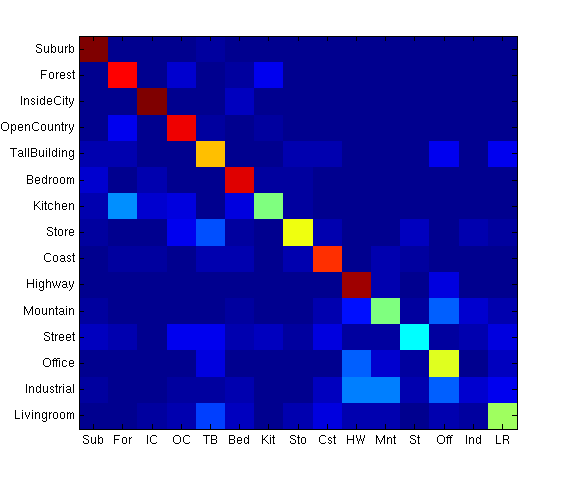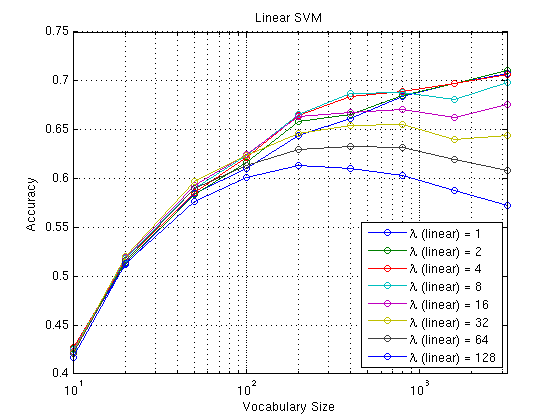Substituting the linear SVM with a radial basis function SVM showed a similar trend. However, the RBF performed better than the linear SVM for lower vocabulary sizes, although the linear SVM started to match and beat the RBF SVM for larger vocabularies. (In the confusion matrix below, gamma of 32 yielded an accuracy of 0.6973 with a vocabulary size of 800)

The radial basis function used was Gaussian where

k(x,y) = \exp(-\gamma ||x-y||^2)

Using Euclidean distance as the distance metric and varying the gamma parameter gave the following result.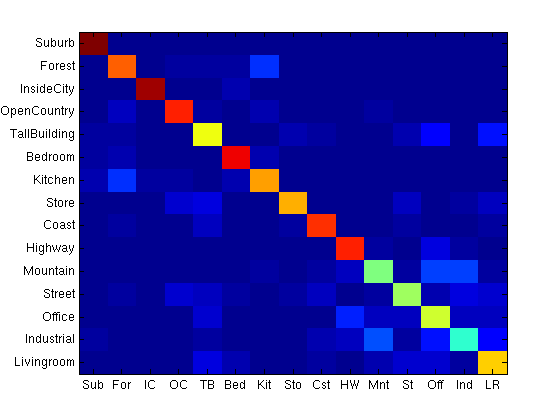A similar experiment was done using a sigmoid kernel. For smaller vocabulary sizes, the kernel matched the performance of the linear kernel, although at larger vocabularies, its performance varied much more.

The sigmoid kernel is defined as

k(x,y) = \tanh(\alpha x^Ty+c)

Alpha was chosen to be 1 over the data dimensionality (128) and the intercept constant c, was varied.

The figure below compares the accuracy versus the vocabulary size for each of the kernel types, and plots the area between the curve of lowest accuracy and the curve of highest accuracy (note that the comparison chart is also plotted on a log scale).

The comparison shows that the RBF generally does better than the linear kernel or sigmoid kernels at lower vocabulary sizes (200-400). As vocabulary size increases, the linear kernel appears to catch up to the other kernels, and eventually starts to surpass the other kernels for very large vocabulary sizes. The wide variance and downturn of the sigmoid kernel as vocabulary size increases may be due to poor free parameter choice, however due to time constraints  this was difficult to fix. These results appear to match (to some extent) those found by Yang, Jiang, Hauptmann, Ngo (2007).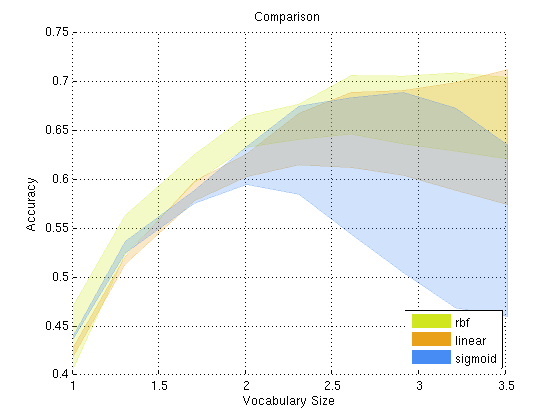For most of the following tests, a linear SVM was used since it uses much less memory and is faster to train and test while not suffering from any performance loss for vocabulary sizes greater than about 800 (based on the above evaluation).

1.2 / Spatial Information

To incorporate spatial information into the image classification process, instead of using different histograms for each portion of an image (as suggested by Lazebnik et al. 2006), the x and y coordinate information were added to the end of the SIFT feature vector, forming a 130-dimensional vector. While simple, the extended feature vector performed better than without spatial information (accuracy of about 0.73 using a vocab size of 800 and RBF with gamma of 64 - figure below left). Using a vocabulary size of 1600 and gamma of 128, accuracy is 0.7653 - these values were chosen since the RBF appears to plateau at around these area in the above analysis (figure below right).This simple extension to the SIFT vector to incorporate spatial information gave surprisingly good results at very little cost or added complexity.

1.3 / Extended Features

Adding and combining other features to the SIFT feature seems to improve performance of the classifier (better than using any one feature by itself - see Xiao, Hays, Ehinger, Oliva, Torralba). Adding on the GIST scene descriptor and LBP (Local binary pattern) features, improved performance to 0.7840, using a linear classifier and vocabulary size of 1600 (see figure below left). These two extra features were chosen since the features they encode do not overlap (that much) with the baseline SIFT descriptor, or with each other. The GIST descriptor aims to encode the general structure of the secene by encoding frequencies, while the LBP feature tries to encode texture information about image regions. For the LBP feature, best peformance seemed to result from using a uniformly sampled rotationally invariant mapping. The LBP code used was taken from http://www.cse.oulu.fi/MVG/Downloads/LBPMatlab, and GIST code was taken from http://people.csail.mit.edu/torralba/code/spatialenvelope/.1.4 / Soft Histogram Binning

Histogram binning is inherently a very lossy operation, since features which are similar may get unlucky and placed into two seperate bins. Instead of simply binning the features into a histogram, a soft binning scheme is used. Following Philbin, Chum, Isard, Sivic, Zisserman (2008), an exponential weighting function over the distance of the k nearest neighbors was chosen, where

w = \exp(-d^2/2\sigma^2)

I slightly modified this weighting function to penalize distances more rapidly, such that

w = \exp(-d^6/2\sigma^2)

This modified weighting function had the slight side effect that sigma^2 had to be very large (around 10e29).

The histogram binning was run using the three neigherest neighbors and weighting each according distance. More neighbors tended to not give any performance gains, since scenes began to become confused with each other.

Soft binning combined with extra features and spatial information and vocabulary size of 800 with a linear SVM gave an accuracy of 0.8027 (figure below left), an improvement of about 1-2%. It might be possible to tune the binning parameters more to get a larger increase in performance.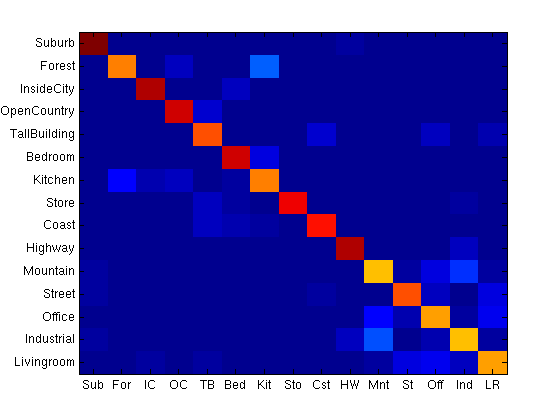1.5 / Cross Validation Results

Final results were evaluated using k-fold cross validation (with k=5). The training and test images were combined and treated as one data set , which was then randomly partitioned into k different subsets. The classifier was then trained on k-1 of the subsets and tested on the last (kth) subset. This process was repeated k times, with the each of the k subsets used exactly once as the test set. Accuracy was taken as the mean of all k runs. k was chosen to be five for cross validation, accuracies are below.

Modifying this schema slightly to ensure 100 training images for a fair comparison (by randomly sampling 100 images again from the k-1 training partitions) and 80-90 test images per class, and evaluating results with a vocab size of 800 gave

>> accuracies

accuracies =

0.8040    0.8412    0.8024    0.7974    0.8174

>> mean(accuracies)

ans =

0.8125

>> var(accuracies)

ans =

3.1345e-04

The confusion matrix (taken as the mean of the k trials) is in the figure below left. To the right is the same confusion matrix, except with the diagonals zeroed out to enhance the contrast between incorrect classifications.

The diagonal of the confusion matrix is

>> diag(conf)'

ans =

Columns 1 through 11

0.9793    0.8278    0.9238    0.8462    0.8214    0.8262    0.7098    0.8664    0.8904    0.9442    0.5926

Columns 12 through 15

0.7749    0.7333    0.6609    0.7905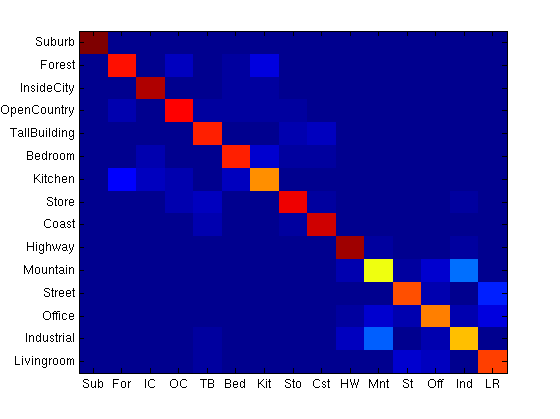More training data can improve performance. If we make use of all of the dataset (200-300) training images per class for each k (standard k-fold cross validation), ie. use all images in the k-1 folds for training instead of just 100 and test on the last, kth fold accuracies improve significantly.

accuracies =

0.8550    0.8520    0.8601    0.8374    0.8558

>> mean(accuracies)

ans =

0.8521

>> var(accuracies)

ans =

7.5507e-05

2 / Notes

It would be interesting to see if the spatial pyramid match kernel gives a significant performance gain over the simple addition of two parameters to each SIFT feature vector - I think it might. It would also be interesting to see how much performance improved by fusing more features (like the SUN database paper), since right now this method is only fusing 3 features together (SIFT, GIST, and LBP), whereas the SUN database paper fuses many more.